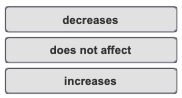# Problem: Which action causes the volume of a gas sample to increase?Match the words in the left column to the appropriate blanks in the sentences on the right.Decreasing the pressure ______ the volume of the gas (at constant temperature and the amount of the gas). Decreasing the temperature _________ the volume of the gas (at constant pressure and the amount of the gas). Decreasing the number of moles _________ the volume of the gas (at constant pressure and temperature).

###### FREE Expert Solution

Decreasing the pressure increases the volume of the gas (at constant temperature and the amount of the gas).

91% (433 ratings)###### Problem Details

Which action causes the volume of a gas sample to increase?

Match the words in the left column to the appropriate blanks in the sentences on the right.

Decreasing the pressure ______ the volume of the gas (at constant temperature and the amount of the gas). Decreasing the temperature _________ the volume of the gas (at constant pressure and the amount of the gas). Decreasing the number of moles _________ the volume of the gas (at constant pressure and temperature).Frequently Asked Questions

What scientific concept do you need to know in order to solve this problem?

Our tutors have indicated that to solve this problem you will need to apply the Chemistry Gas Laws concept. You can view video lessons to learn Chemistry Gas Laws. Or if you need more Chemistry Gas Laws practice, you can also practice Chemistry Gas Laws practice problems.

What professor is this problem relevant for?

Based on our data, we think this problem is relevant for Professor Guerra's class at RUTGERS.